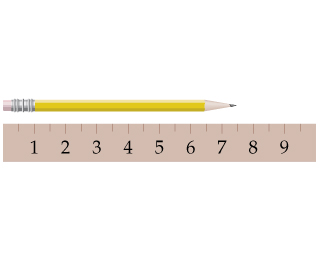# Problem: What is the length of the pencil in the figure if the scale reads in centimeters?

###### FREE Expert Solution

We have to determine the length of the pencil placed next to a scale that reads in centimeters.

We can see that the numbers on the scale are in the increments of 1 and the lines halfway between two digits have values halfway between them.

94% (492 ratings)###### Problem Details

What is the length of the pencil in the figure if the scale reads in centimeters?